Next: Implementation Up: Brent method for solving Previous: Brent method for solving   Contents

Mathematical background

Brent method is an iterative scheme used to obtain one root of the equation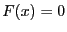within an interval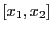. It assumes that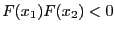. Let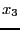be the mid-point of the interval. A new estimate of the root is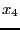with: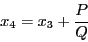with: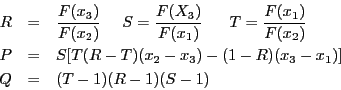In this methodis considered to be the current estimate of the solution. The term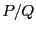is a correction factor: when this factor leads to a new estimate of the solution outside the interval we use a bisection method to compute a new interval. In other words if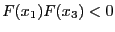the new interval is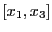and if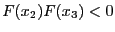the new interval is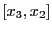. Therefore Brent method is a cross between a bisection method and a super-linear method which insure that the estimate of the solution always lie within the interval.

Jean-Pierre Merlet 2012-12-20